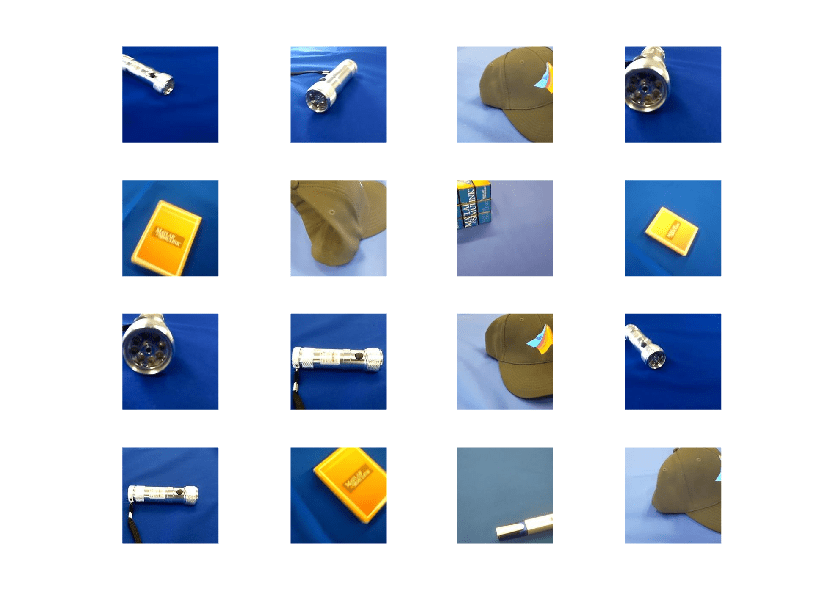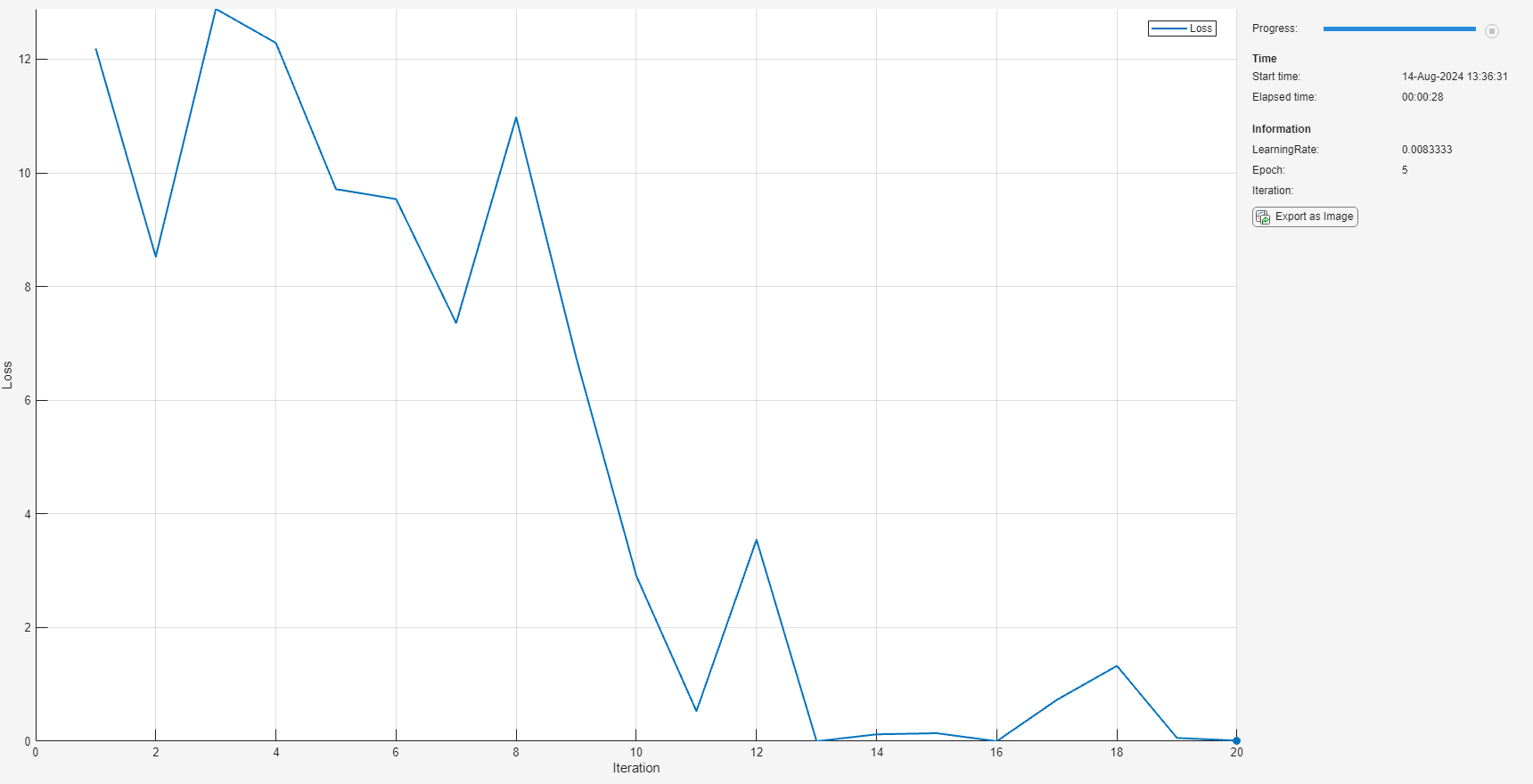Main Content

# ONNXParameters

Parameters of imported ONNX network for deep learning

## Description

`ONNXParameters` contains the parameters (such as weights and bias) of an imported ONNX™ (Open Neural Network Exchange) network. Use `ONNXParameters` to perform tasks such as transfer learning.

## Creation

Create an `ONNXParameters` object by using `importONNXFunction`.

## Properties

expand all

Parameters updated during network training, specified as a structure. For example, the weights of convolution and fully connected layers are parameters that the network learns during training. To prevent `Learnables` parameters from being updated during training, convert them to `Nonlearnables` by using `freezeParameters`. Convert frozen parameters back to `Learnables` by using `unfreezeParameters`.

Add a new parameter to `params.Learnables` by using `addParameter`. Remove a parameter from `params.Learnables` by using `removeParameter`.

Access the fields of the structure `Learnables` by using dot notation. For example, `params.Learnables.conv1_W` could display the weights of the first convolution layer. Initialize the weights for transfer learning by entering `params.Learnables.conv1_W = rand([1000,4096])`. For more details about assigning a new value and parameter naming, see Tips.

Parameters unchanged during network training, specified as a structure. For example, padding and stride are parameters that stay constant during training.

Add a new parameter to `params.Nonlearnables` by using `addParameter`. Remove a parameter from `params.Nonlearnables` by using `removeParameter`.

Access the fields of the structure `Nonlearnables` by using dot notation. For example, `params.Nonlearnables.conv1_Padding` could display the padding of the first convolution layer. For more details about parameter naming, see Tips.

Network state, specified as a structure. The network `State` contains information remembered by the network between iterations and updated across multiple training batches. For example, the states of LSTM and batch normalization layers are `State` parameters.

Add a new parameter to `params.State` by using `addParameter`. Remove a parameter from `params.State` by using `removeParameter`.

Access the fields of the structure `State` by using dot notation. For example, `params.State.bn1_var` could display the variance of the first batch normalization layer. For more details about parameter naming, see Tips.

This property is read-only.

Number of dimensions for every parameter, specified as a structure. `NumDimensions` includes trailing singleton dimensions.

Access the fields of the structure `NumDimensions` by using dot notation. For example, `params.NumDimensions.conv1_W` could display the number of dimensions for the weights parameter of the first convolution layer.

This property is read-only.

Name of the model function, specified as a character vector or string scalar. The property `NetworkFunctionName` contains the name of the function `NetworkFunctionName`, which you specify in `importONNXFunction`. The function `NetworkFunctionName` contains the architecture of the imported ONNX network.

Example: `'shufflenetFcn'`

## Object Functions

 `addParameter` Add parameter to `ONNXParameters` object `freezeParameters` Convert learnable network parameters in `ONNXParameters` to nonlearnable `removeParameter` Remove parameter from `ONNXParameters` object `unfreezeParameters` Convert nonlearnable network parameters in `ONNXParameters` to learnable

## Examples

collapse all

Import the `squeezenet` convolution neural network as a function and fine-tune the pretrained network with transfer learning to perform classification on a new collection of images.

This example uses several helper functions. To view the code for these functions, see Helper Functions.

Unzip and load the new images as an image datastore. `imageDatastore` automatically labels the images based on folder names and stores the data as an `ImageDatastore` object. An image datastore enables you to store large image data, including data that does not fit in memory, and efficiently read batches of images during training of a convolutional neural network. Specify the mini-batch size.

```unzip('MerchData.zip'); miniBatchSize = 8; imds = imageDatastore('MerchData', ... 'IncludeSubfolders',true, ... 'LabelSource','foldernames',... 'ReadSize', miniBatchSize);```

This data set is small, containing 75 training images. Display some sample images.

```numImages = numel(imds.Labels); idx = randperm(numImages,16); figure for i = 1:16 subplot(4,4,i) I = readimage(imds,idx(i)); imshow(I) end```Extract the training set and one-hot encode the categorical classification labels.

```XTrain = readall(imds); XTrain = single(cat(4,XTrain{:})); YTrain_categ = categorical(imds.Labels); YTrain = onehotencode(YTrain_categ,2)';```

Determine the number of classes in the data.

```classes = categories(YTrain_categ); numClasses = numel(classes)```
```numClasses = 5 ```

`squeezenet` is a convolutional neural network that is trained on more than a million images from the ImageNet database. As a result, the network has learned rich feature representations for a wide range of images. The network can classify images into 1000 object categories, such as keyboard, mouse, pencil, and many animals.

Import the pretrained `squeezenet` network as a function.

```squeezenetONNX() params = importONNXFunction('squeezenet.onnx','squeezenetFcn')```
```A function containing the imported ONNX network has been saved to the file squeezenetFcn.m. To learn how to use this function, type: help squeezenetFcn. ```
```params = ONNXParameters with properties: Learnables: [1×1 struct] Nonlearnables: [1×1 struct] State: [1×1 struct] NumDimensions: [1×1 struct] NetworkFunctionName: 'squeezenetFcn' ```

`params` is an `ONNXParameters` object that contains the network parameters. `squeezenetFcn` is a model function that contains the network architecture. `importONNXFunction` saves `squeezenetFcn` in the current folder.

Calculate the classification accuracy of the pretrained network on the new training set.

```accuracyBeforeTraining = getNetworkAccuracy(XTrain,YTrain,params); fprintf('%.2f accuracy before transfer learning\n',accuracyBeforeTraining);```
```0.01 accuracy before transfer learning ```

The accuracy is very low.

Display the learnable parameters of the network by typing `params.Learnables`. These parameters, such as the weights (`W`) and bias (`B`) of convolution and fully connected layers, are updated by the network during training. Nonlearnable parameters remain constant during training.

The last two learnable parameters of the pretrained network are configured for 1000 classes.

`conv10_W: [1×1×512×1000 dlarray]`

`conv10_B: [1000×1 dlarray]`

The parameters `conv10_W` and `conv10_B` must be fine-tuned for the new classification problem. Transfer the parameters to classify five classes by initializing the parameters.

```params.Learnables.conv10_W = rand(1,1,512,5); params.Learnables.conv10_B = rand(5,1);```

Freeze all the parameters of the network to convert them to nonlearnable parameters. Because you do not need to compute the gradients of the frozen layers, freezing the weights of many initial layers can significantly speed up network training.

`params = freezeParameters(params,'all');`

Unfreeze the last two parameters of the network to convert them to learnable parameters.

```params = unfreezeParameters(params,'conv10_W'); params = unfreezeParameters(params,'conv10_B');```

Now the network is ready for training. Initialize the training progress plot.

```plots = "training-progress"; if plots == "training-progress" figure lineLossTrain = animatedline; xlabel("Iteration") ylabel("Loss") end```

Specify the training options.

```velocity = []; numEpochs = 5; miniBatchSize = 16; numObservations = size(YTrain,2); numIterationsPerEpoch = floor(numObservations./miniBatchSize); initialLearnRate = 0.01; momentum = 0.9; decay = 0.01;```

Train the network.

```iteration = 0; start = tic; executionEnvironment = "cpu"; % Change to "gpu" to train on a GPU. % Loop over epochs. for epoch = 1:numEpochs % Shuffle data. idx = randperm(numObservations); XTrain = XTrain(:,:,:,idx); YTrain = YTrain(:,idx); % Loop over mini-batches. for i = 1:numIterationsPerEpoch iteration = iteration + 1; % Read mini-batch of data. idx = (i-1)*miniBatchSize+1:i*miniBatchSize; X = XTrain(:,:,:,idx); Y = YTrain(:,idx); % If training on a GPU, then convert data to gpuArray. if (executionEnvironment == "auto" && canUseGPU) || executionEnvironment == "gpu" X = gpuArray(X); end % Evaluate the model gradients and loss using dlfeval and the % modelGradients function. [gradients,loss,state] = dlfeval(@modelGradients,X,Y,params); params.State = state; % Determine the learning rate for the time-based decay learning rate schedule. learnRate = initialLearnRate/(1 + decay*iteration); % Update the network parameters using the SGDM optimizer. [params.Learnables,velocity] = sgdmupdate(params.Learnables,gradients,velocity); % Display the training progress. if plots == "training-progress" D = duration(0,0,toc(start),'Format','hh:mm:ss'); addpoints(lineLossTrain,iteration,double(gather(extractdata(loss)))) title("Epoch: " + epoch + ", Elapsed: " + string(D)) drawnow end end end```Calculate the classification accuracy of the network after fine-tuning.

```accuracyAfterTraining = getNetworkAccuracy(XTrain,YTrain,params); fprintf('%.2f accuracy after transfer learning\n',accuracyAfterTraining);```
```1.00 accuracy after transfer learning ```

Helper Functions

This section provides the code of the helper functions used in this example.

The `getNetworkAccuracy` function evaluates the network performance by calculating the classification accuracy.

```function accuracy = getNetworkAccuracy(X,Y,onnxParams) N = size(X,4); Ypred = squeezenetFcn(X,onnxParams,'Training',false); [~,YIdx] = max(Y,[],1); [~,YpredIdx] = max(Ypred,[],1); numIncorrect = sum(abs(YIdx-YpredIdx) > 0); accuracy = 1 - numIncorrect/N; end```

The `modelGradients` function calculates the loss and gradients.

```function [grad, loss, state] = modelGradients(X,Y,onnxParams) [y,state] = squeezenetFcn(X,onnxParams,'Training',true); loss = crossentropy(y,Y,'DataFormat','CB'); grad = dlgradient(loss,onnxParams.Learnables); end```

The `squeezenetONNX` function generates an ONNX model of the `squeezenet` network.

```function squeezenetONNX() exportONNXNetwork(squeezenet,'squeezenet.onnx'); end ```

Import a network saved in the ONNX format as a function, and move the mislabeled parameters by using `freeze` or `unfreeze`.

Import the pretrained `simplenet.onnx` network as a function. `simplenet` is a simple convolutional neural network trained on digit image data. For more information on how to create `simplenet`, see Create Simple Image Classification Network.

Import `simplenet.onnx` using `importONNXFunction`, which returns an `ONNXParameters` object that contains the network parameters. The function also creates a new model function in the current folder that contains the network architecture. Specify the name of the model function as `simplenetFcn`.

`params = importONNXFunction('simplenet.onnx','simplenetFcn');`
```A function containing the imported ONNX network has been saved to the file simplenetFcn.m. To learn how to use this function, type: help simplenetFcn. ```

`importONNXFunction` labels the parameters of the imported network as `Learnables` (parameters that are updated during training) or `Nonlearnables` (parameters that remain unchanged during training). The labeling is not always accurate. A recommended practice is to check if the parameters are assigned to the correct structure `params.Learnables` or `params.Nonlearnables`. Display the learnable and nonlearnable parameters of the imported network.

`params.Learnables`
```ans = struct with fields: imageinput_Mean: [1×1 dlarray] conv_W: [5×5×1×20 dlarray] conv_B: [20×1 dlarray] batchnorm_scale: [20×1 dlarray] batchnorm_B: [20×1 dlarray] fc_W: [24×24×20×10 dlarray] fc_B: [10×1 dlarray] ```
`params.Nonlearnables`
```ans = struct with fields: ConvStride1004: [2×1 dlarray] ConvDilationFactor1005: [2×1 dlarray] ConvPadding1006: [4×1 dlarray] ConvStride1007: [2×1 dlarray] ConvDilationFactor1008: [2×1 dlarray] ConvPadding1009: [4×1 dlarray] ```

Note that `params.Learnables` contains the parameter `imageinput_Mean`, which should remain unchanged during training (see the `Mean` property of `imageInputLayer`). Convert `imageinput_Mean` to a nonlearnable parameter. The `freezeParameters` function removes the parameter `imageinput_Mean` from `param.Learnables` and adds it to `params.Nonlearnables` sequentially.

`params = freezeParameters(params,'imageinput_Mean');`

Display the updated learnable and nonlearnable parameters.

`params.Learnables`
```ans = struct with fields: conv_W: [5×5×1×20 dlarray] conv_B: [20×1 dlarray] batchnorm_scale: [20×1 dlarray] batchnorm_B: [20×1 dlarray] fc_W: [24×24×20×10 dlarray] fc_B: [10×1 dlarray] ```
`params.Nonlearnables`
```ans = struct with fields: ConvStride1004: [2×1 dlarray] ConvDilationFactor1005: [2×1 dlarray] ConvPadding1006: [4×1 dlarray] ConvStride1007: [2×1 dlarray] ConvDilationFactor1008: [2×1 dlarray] ConvPadding1009: [4×1 dlarray] imageinput_Mean: [1×1 dlarray] ```

## Tips

• The following rules apply when you assign a new value to a `params.Learnables` parameter:

• The software automatically converts the new value to a `dlarray`.

• The new value must be compatible with the existing value of `params.NumDimensions`.

• `importONNXFunction` derives the field names of the structures `Learnables`, `Nonlearnables`, and `State` from the names in the imported ONNX model file. The field names might differ between imported networks.

## See Also

### Topics

Introduced in R2020b

## Support

#### Introducing Deep Learning with MATLAB

Download ebook